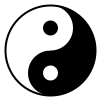# Example 2: Taijitu (Yin Yang)

```// Processing.js example by Jim Bumgardner

size(200,200);
smooth();
background(255);

noStroke();

float diam = width*.9;
float cx = width/2;
float cy = height/2;
float sm_diam = diam/2.0;
float cx1 = cx;
float cy1 = cy + sm_rad;
float cx2 = cx;
float cy2 = cy - sm_rad;

fill(0);
ellipse(cx,cy,diam,diam);

fill(255); // we use a white rectangle to blank out half the circle
rect(0,0,width/2,height);

fill(0);
ellipse(cx1,cy1,sm_diam,sm_diam);

fill(255);
ellipse(cx2,cy2,sm_diam,sm_diam);
ellipse(cx1,cy1,sm_diam/3,sm_diam/3);

fill(0);
ellipse(cx2,cy2,sm_diam/3,sm_diam/3);

stroke(0);
noFill();
strokeWeight(2);
ellipse(cx,cy,diam,diam);

```

Let's examine the program in detail. First there is some basic set-up stuff. I set the size of the drawing to 200 x 200, turn on smoothing, and paint the background white. I turn off stroking so that the white shapes I draw won't have black outlines around them.

```  size(200, 200);
smooth();
background(255);

noStroke();
```
Then I compute the diameter of the outer circle, and its center cordinates, based on the overall width of the drawing. I make it proportional to the width so that if the size() is changed, the figure will resize along with the drawing.
```  float diam = width*.9;
float cx = width/2;
float cy = height/2;
```
The final figure contains curves which are based on a circle exactly half the size of the larger circle. Here, I work out the diameter and radius of this smaller circle.
```  float sm_diam = diam/2.0;
```
Then I work out coordinates for the center of the black and white dots in the figure.
```  float cx1 = cx;
float cy1 = cy + sm_rad;
float cx2 = cx;
float cy2 = cy - sm_rad;
```
Now I am ready to start drawing. First I draw a large black circle.

```  fill(0);
ellipse(cx,cy,diam,diam);
```
It looks like this: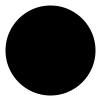Then, I paint half the canvas with a white rectangle, to blank out half of the circle.

``` fill(255); // we use a white rectangle to blank out half the circle
rect(0,0,width/2,height);
```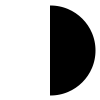Then I draw a small black half-sized circle.

```  fill(0);
ellipse(cx1,cy1,sm_diam,sm_diam);
```And a small white circle above it.

```  fill(255);
ellipse(cx2,cy2,sm_diam,sm_diam);
```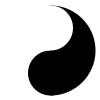Then the small white eye. The fill color is still white, so I don't need to re-specify it. The diameter of the small white eye is 1/3rd that of the half-sized circle (I find a size I liked through experimentation, the figures I've found online vary depending on the artist).

```  ellipse(cx1,cy1,sm_diam/3,sm_diam/3);
```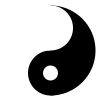Then the small black eye.

```  fill(0);
ellipse(cx2,cy2,sm_diam/3,sm_diam/3);
```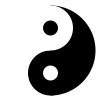Finally, I am ready to draw the circular frame. I use a slightly thicker line thickness of 2 here, because I think it looks nice.

```  stroke(0);
noFill();
strokeWeight(2);
ellipse(cx,cy,diam,diam);
```